# 二元气体混合层的稳定性分析 *

(1. 中国航天空气动力技术研究院，北京 100074； 2. 唐山学院 基础教学部， 河北 唐山 063000； 3. 北京动力机械研究所， 北京 100074)

## 1 控 制 方 程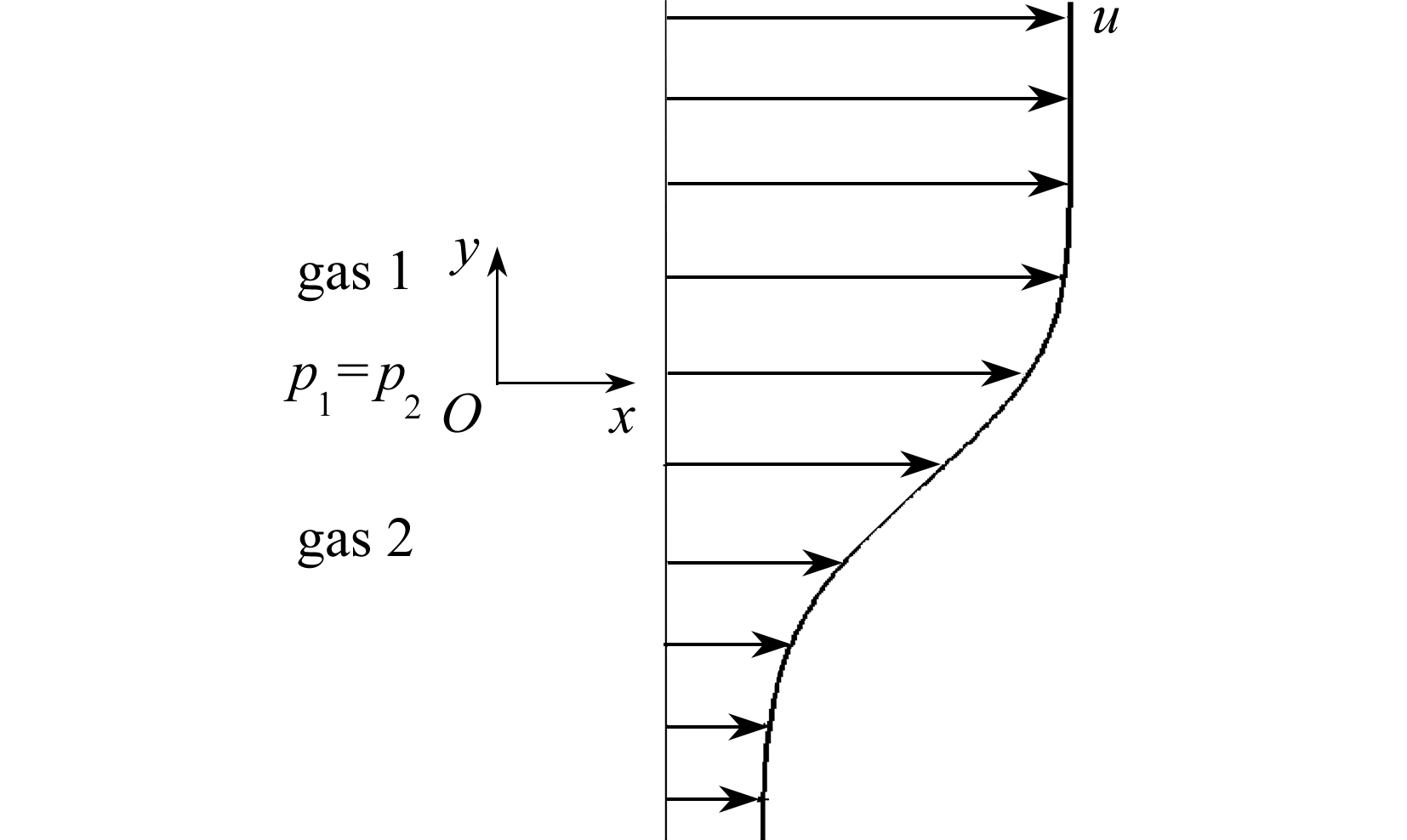Fig. 1 Schematic diagram of binary gas mixing layers

### 1 . 1 相似性解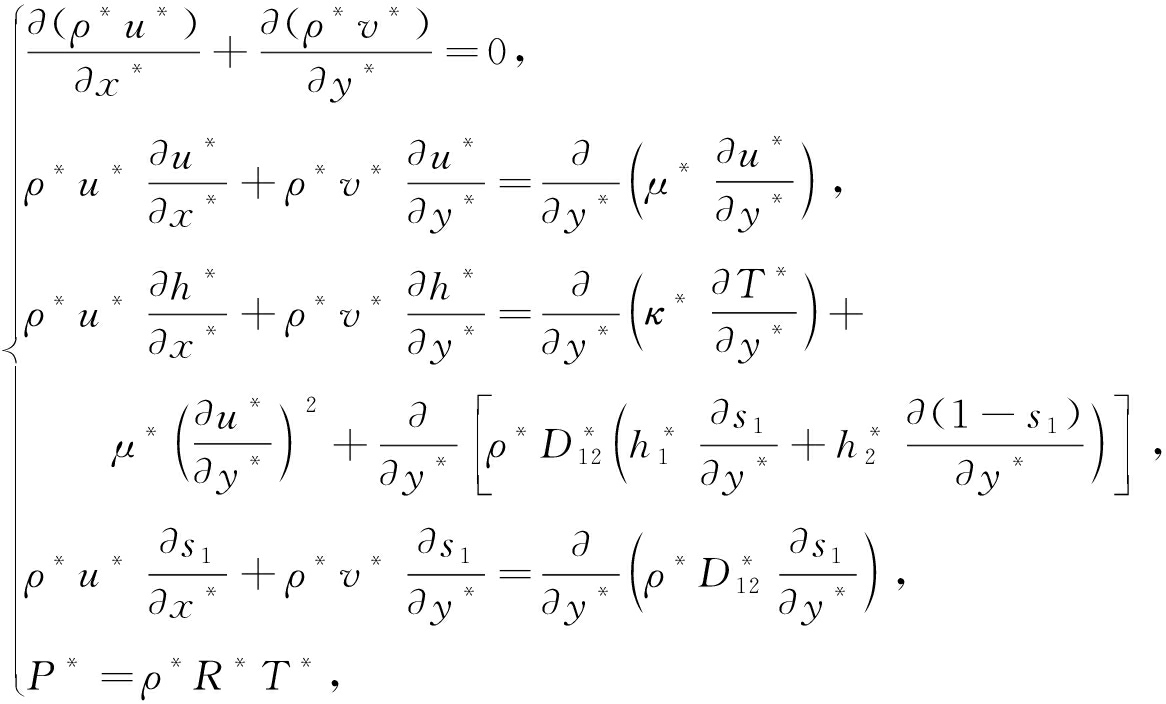(1)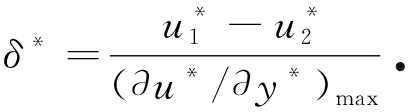(2)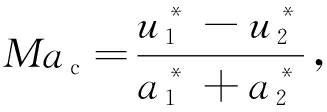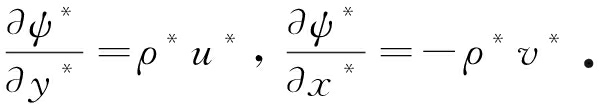(3)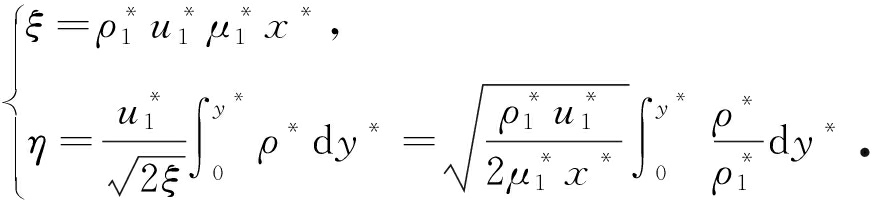(4)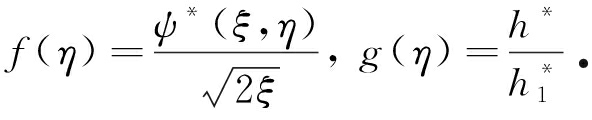(5)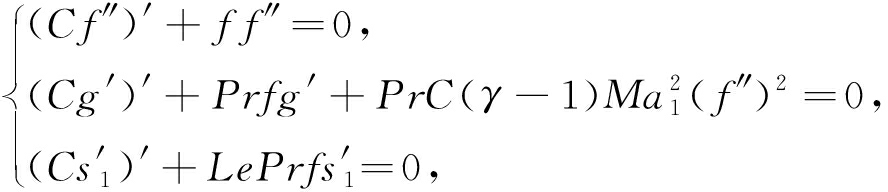(6)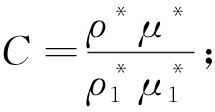(7)

Le 为Lewis数：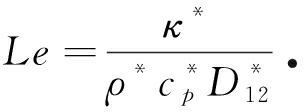(8)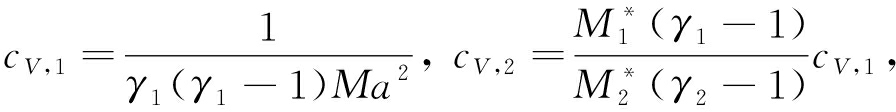(9)

c p ,1 = γ 1 c V ,1 , c p ,2 = γ 2 c V ,2 ,

(10)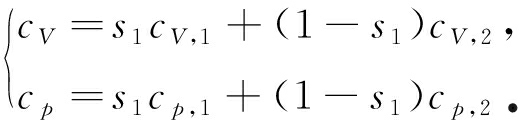(11)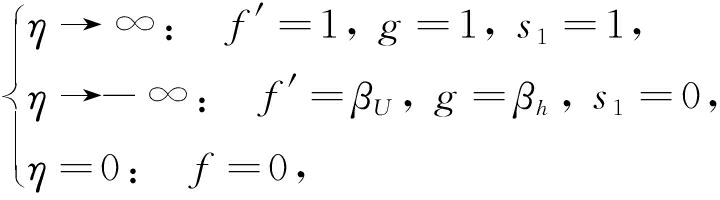(12)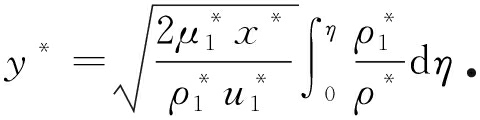(13)

y * 无量纲化后有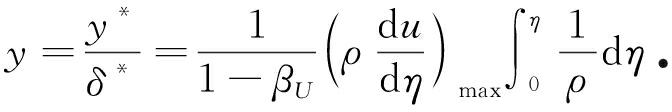(14)

η 坐标下的物理量转换到 y 坐标下，可以得到用相似性解表示的基本流

### 1 . 2 稳定性方程

φ = φ 0 + φ

(15)

L(∂ t ,∂ x ,∂ y ,∂ z ) φ ′=0

(16)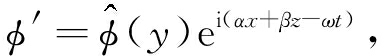(17)(18)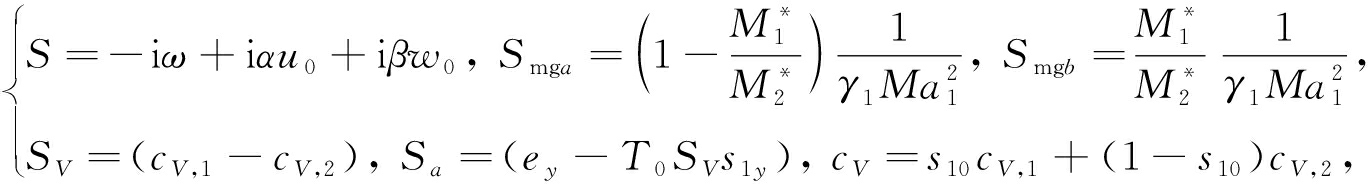(19)

e y 为气体内能 e 关于 y 的导数

Table 1 Thermal properties of O 2 , H 2 and N 2 at 1 atm and 300 K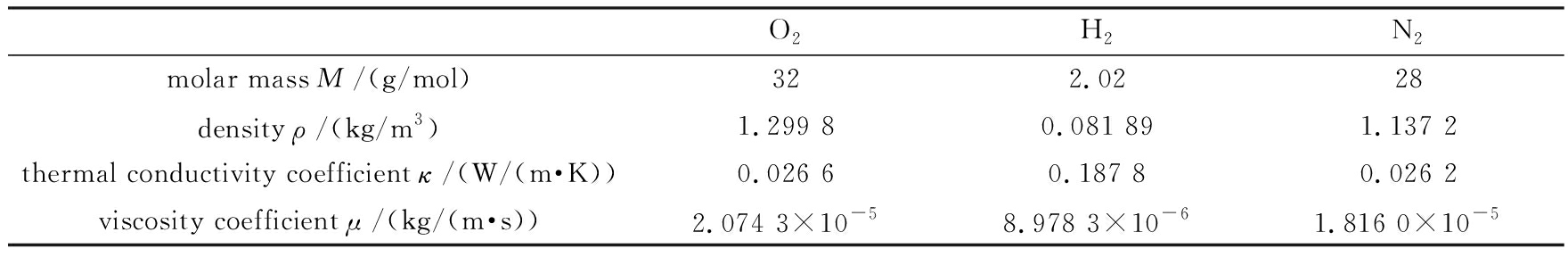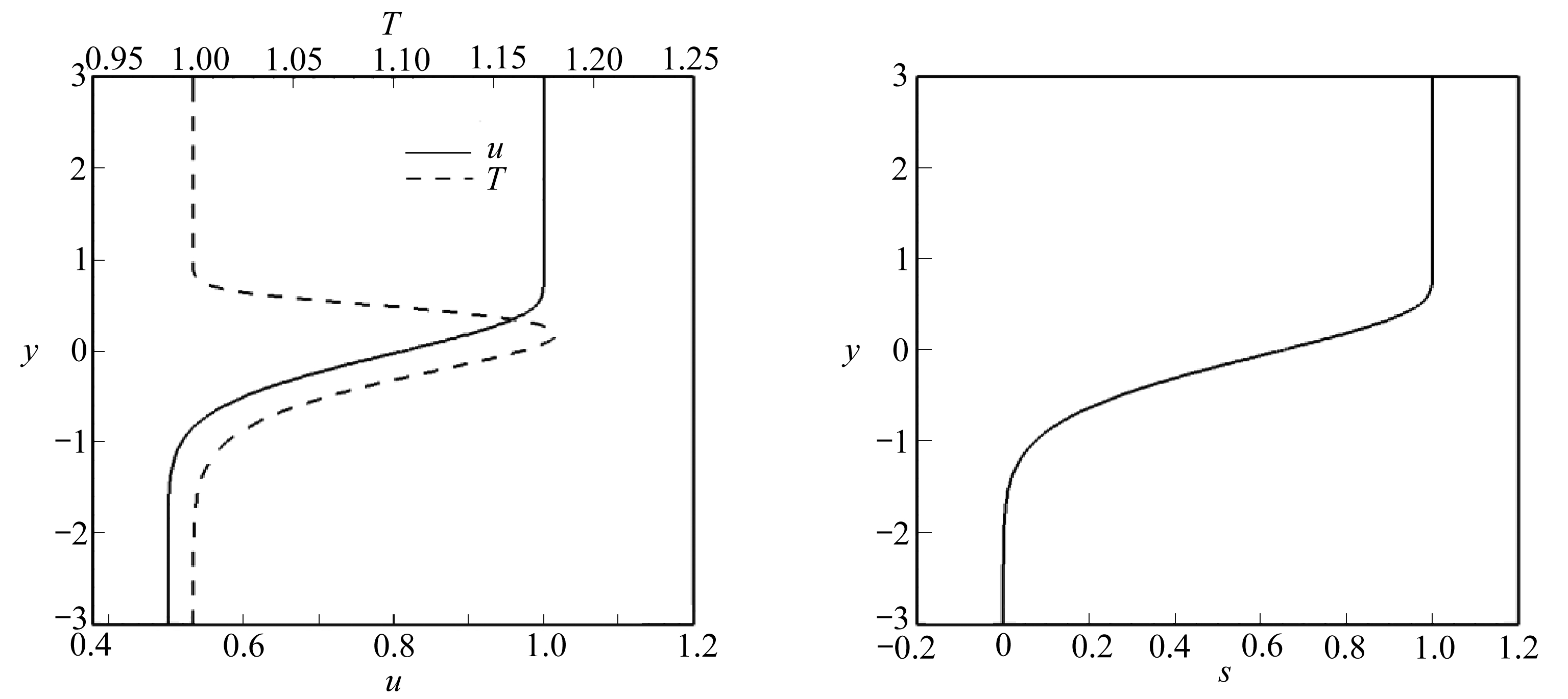(a) 速度和温度剖面 (b) 氧气的质量分数剖面
(a) The profile of the velocity and the temperature (b) The profile of the O 2 mass fraction

Fig. 2 Similar solutions of mixing layers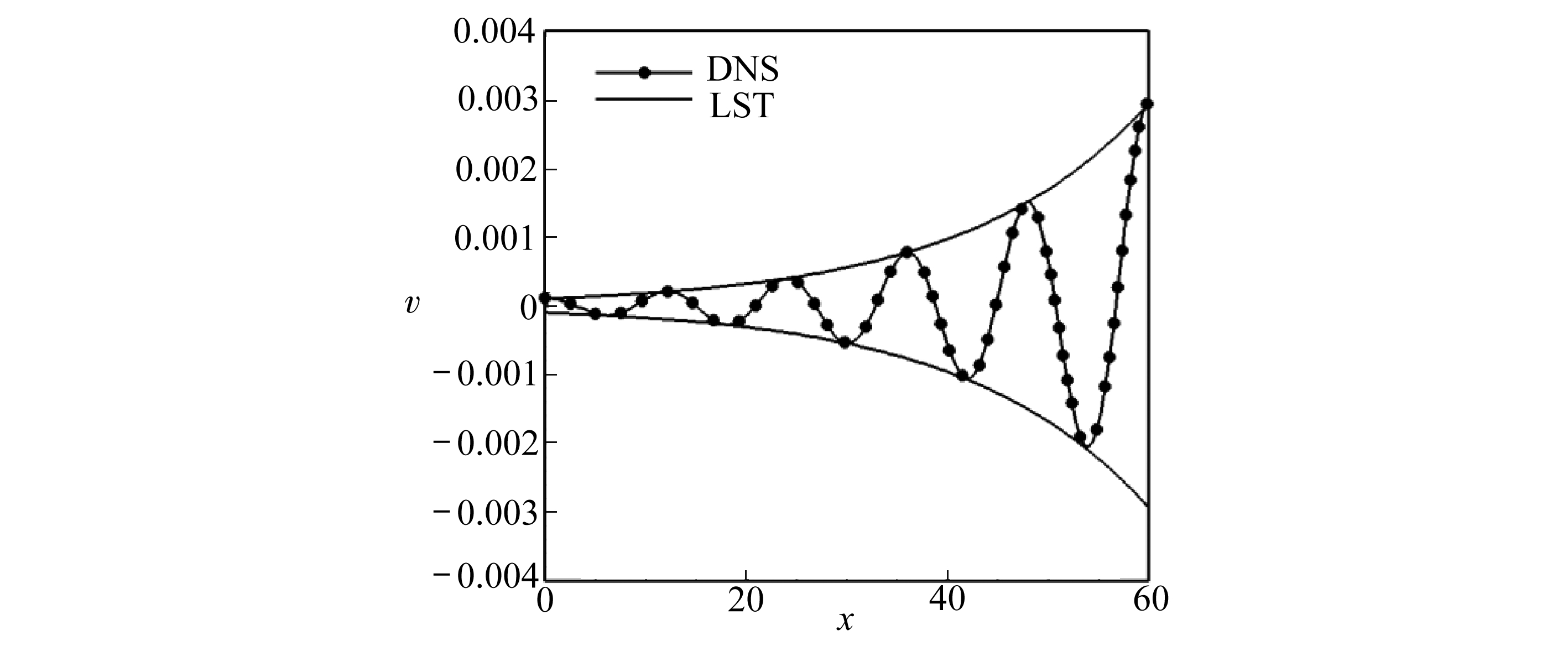Fig. 3 Comparison of the results of DNS and LST

## 2 结 果

Table 2 Convective Mach numbers and corresponding Mach numbers of O 2 -N 2 mixing layers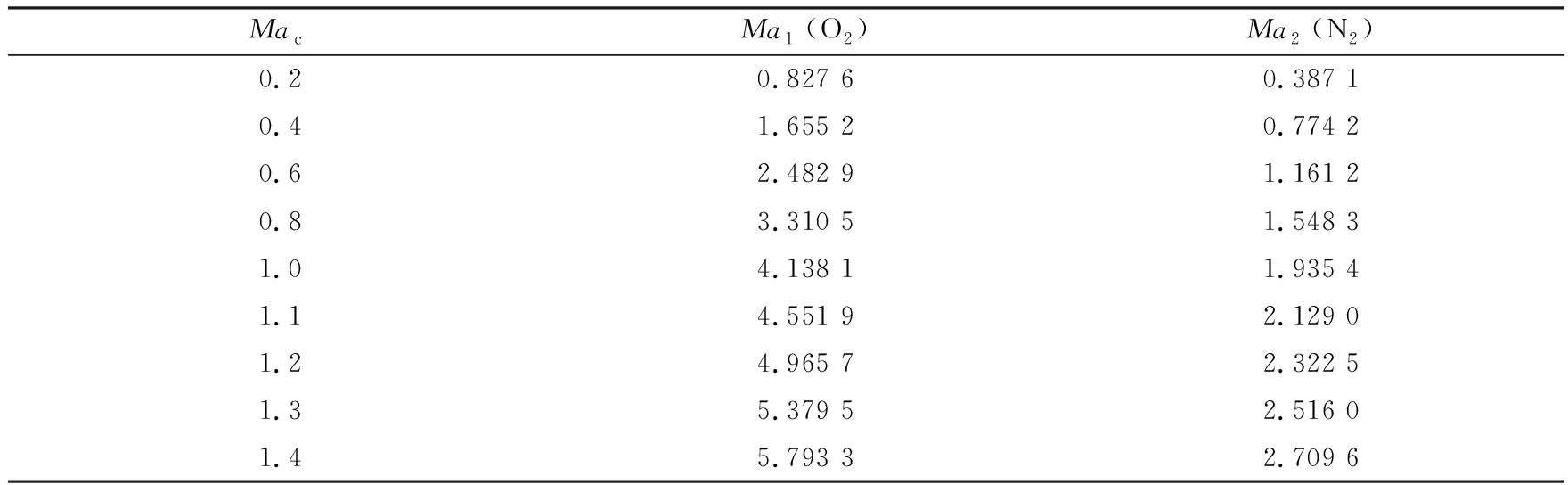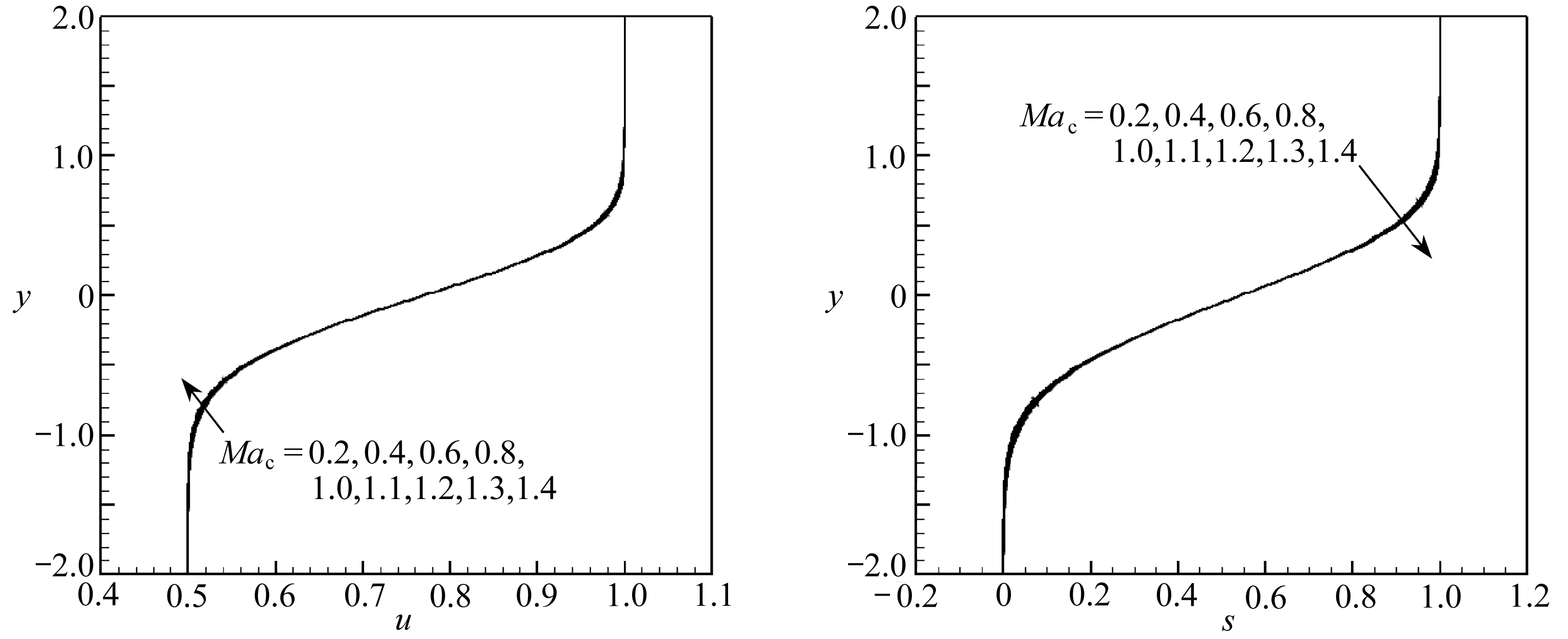(a) 速度 (b) O 2 的质量分数
(a) Velocity (b) The mass fraction of O 2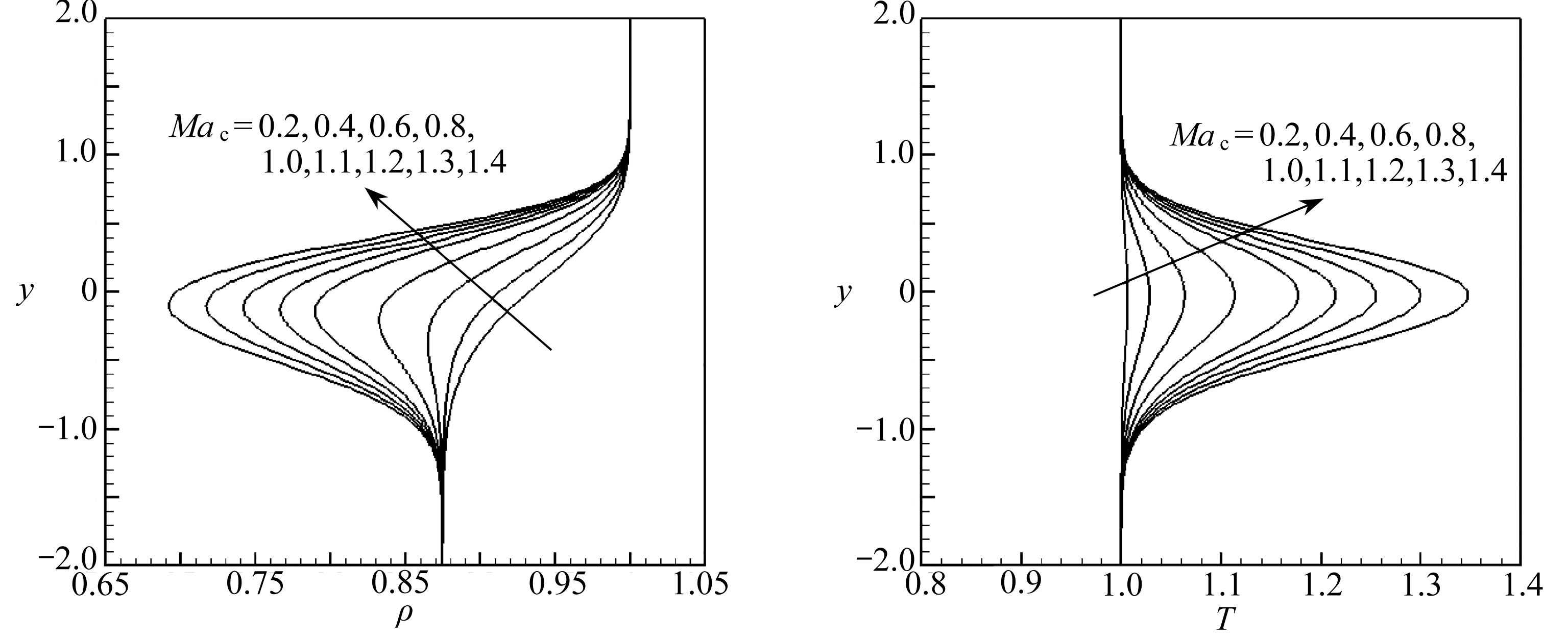(c)密度 (d)温度
(c) Density (d) Temperature

Fig. 4 Similar solution profile with different convective Mach number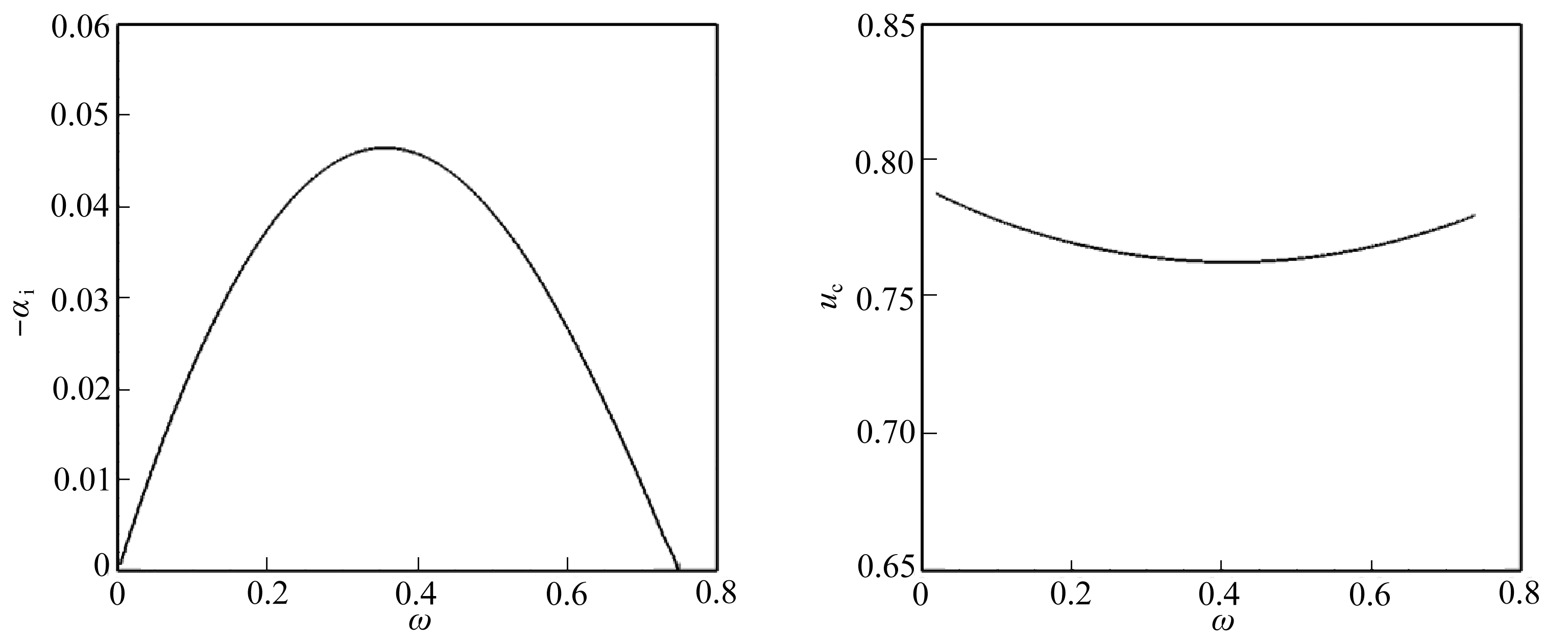(a) 增长率 (b) 相速度
(a) The growth rate (b) The phase speed

Fig. 5 The growth rate and the phase speed vs. the frequency for 2D disturbance at Ma c =0.8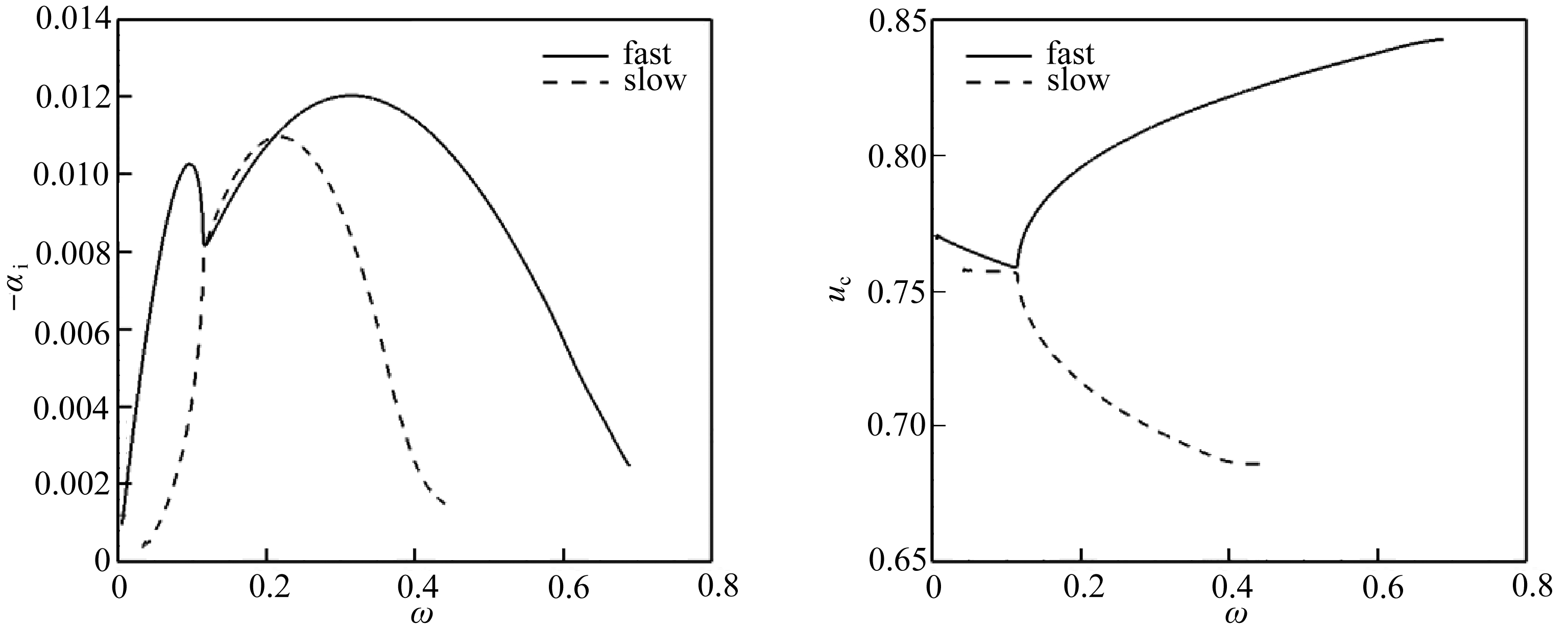(a) 增长率 (b) 相速度
(a) The growth rate (b) The phase speed

Fig. 6 The growth rate and the phase speed vs. the frequency for fast and slow modes at Ma c =1.1

Table 3 Eigenvalues of the 1st mode at Ma c =0.8,1.1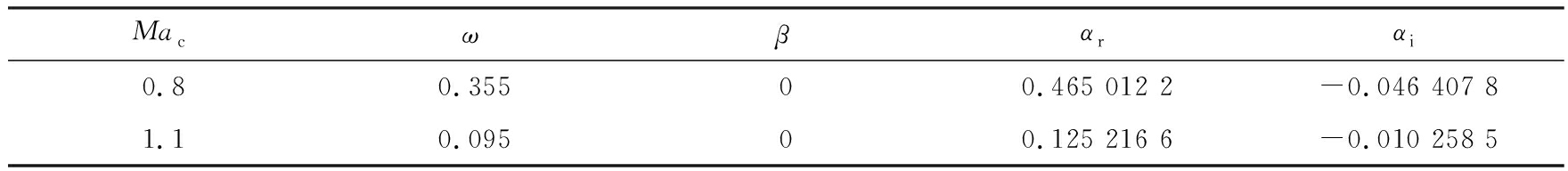Table 4 Eigenvalues of fast and slow modes at Ma c =1.1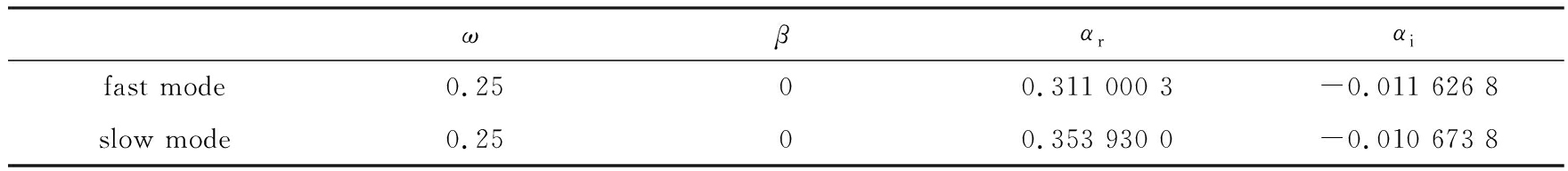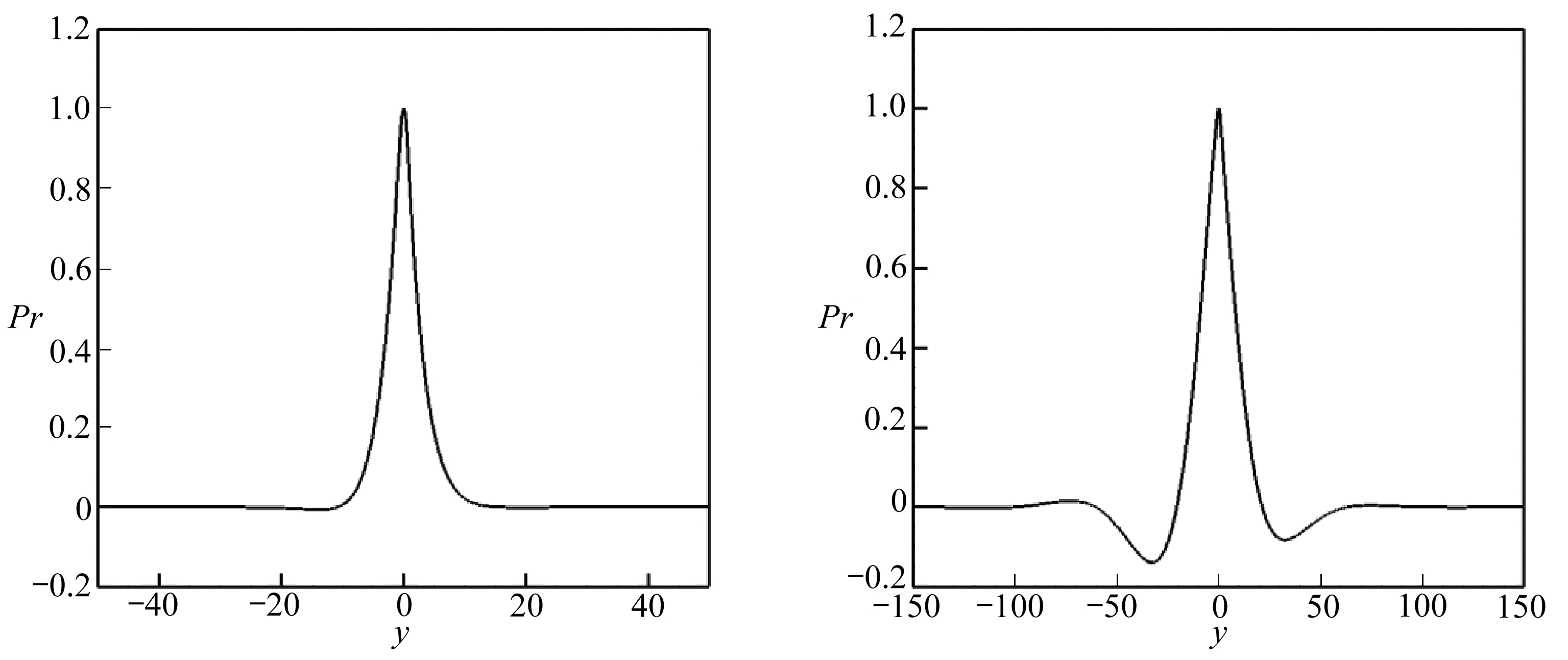(a) Ma c =0.8 (b) Ma c =1.1

Fig. 7 Eigenfunction profiles of the disturbance pressure at different convective Mach numbers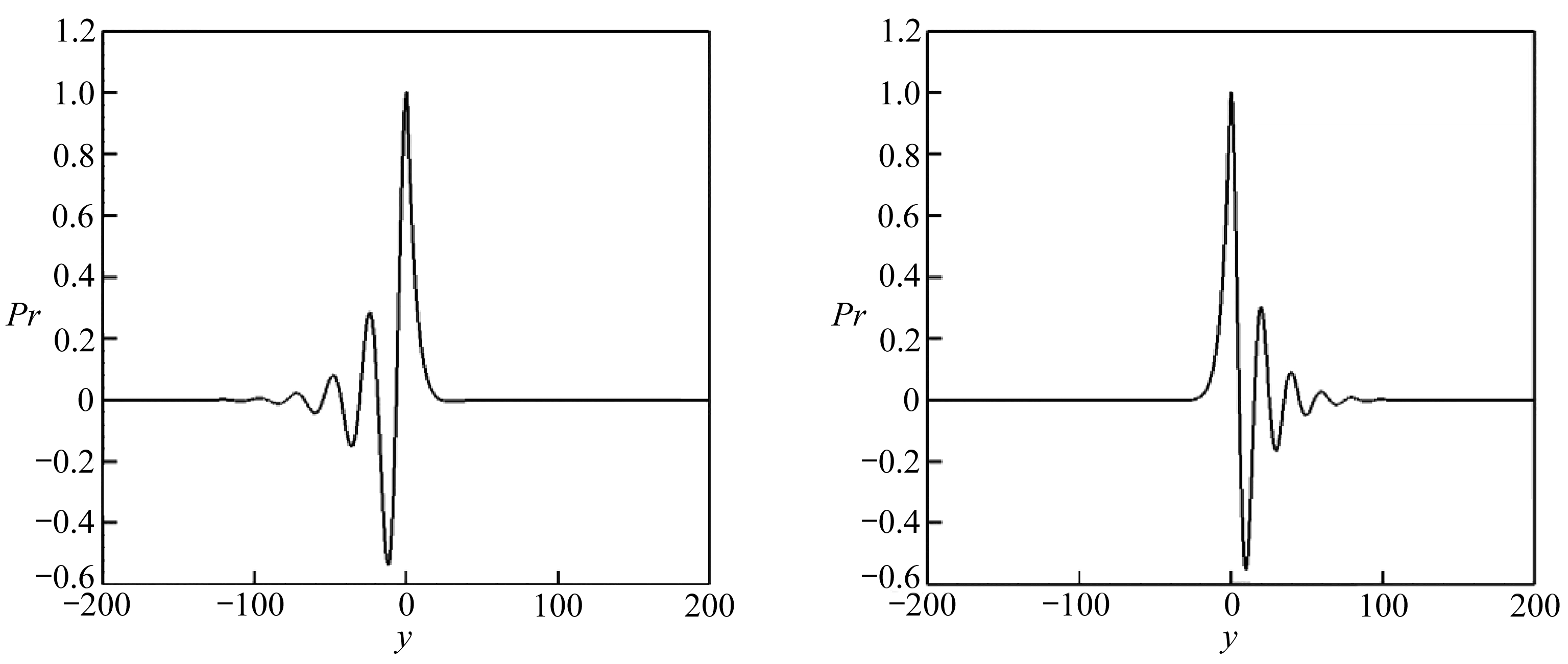(a) 快模态 (b) 慢模态
(a) The fast mode (b) The slow mode

Fig. 8 Eigenfunction profiles of the disturbance pressure of fast and slow modes at Ma c =1.1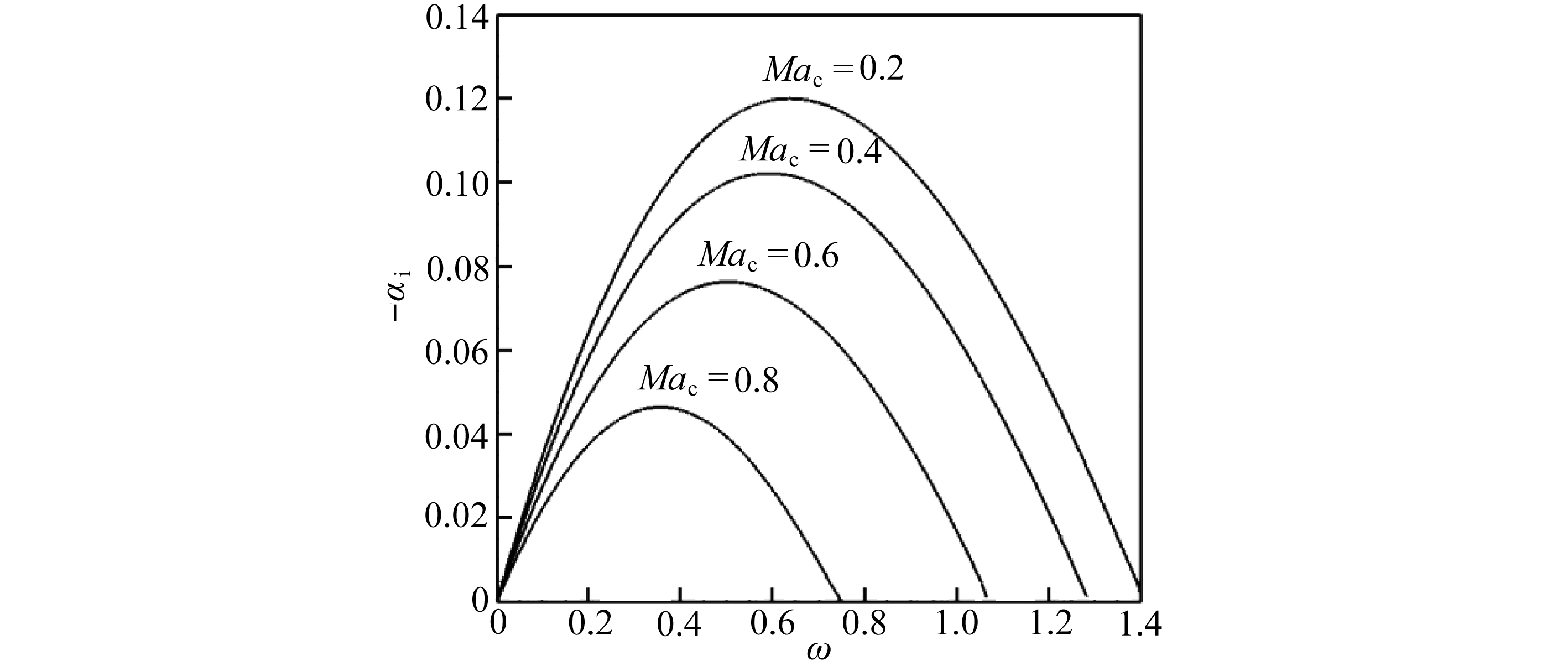Fig. 9 The growth rate at different convective Mach numbers, Ma c <1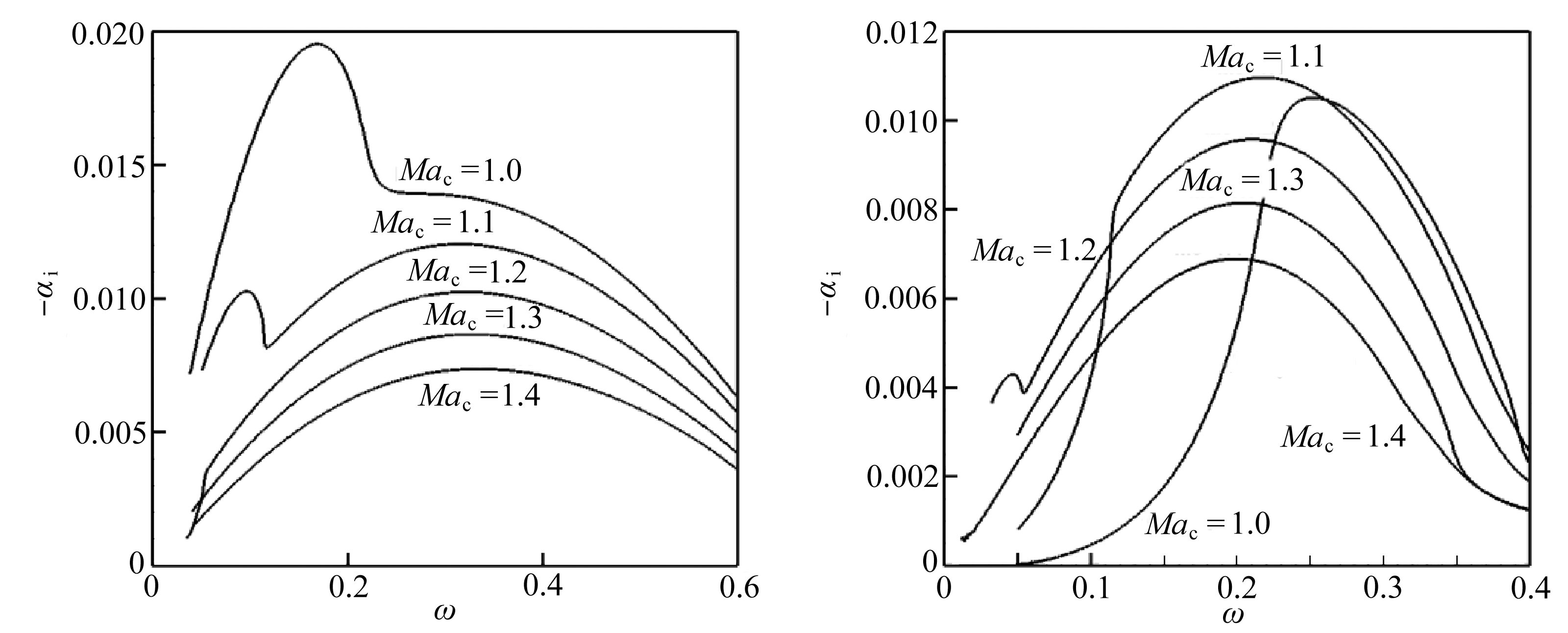(a) 快模态 (b) 慢模态
(a) The fast mode (b) The slow mode

Fig. 10 The growth rates of fast and slow modes at different convective Mach numbers, Ma c ≥1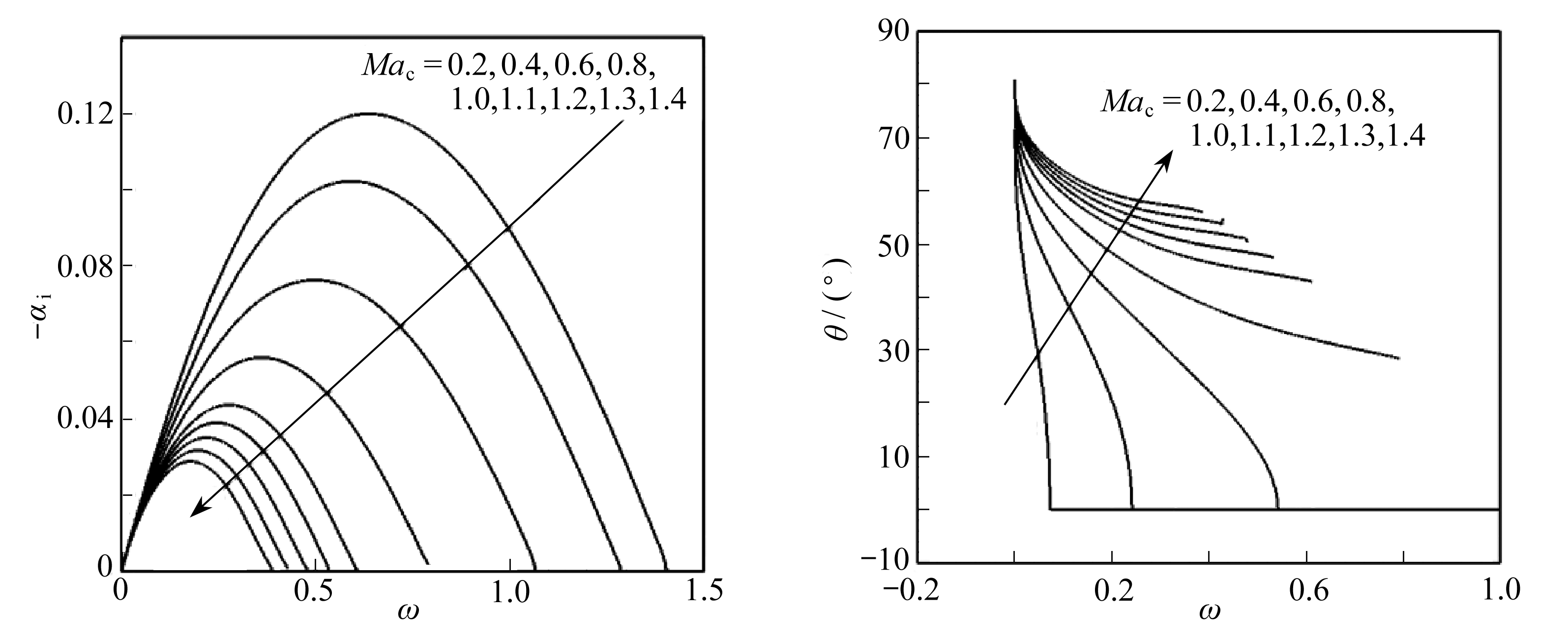Fig. 11 The maximum growth rates of the 1st mode Fig. 12 The wave angles of the most unstable wave vs. the frequency at different convective of the 1st mode vs. the frequency at Mach numbers different Mach numbers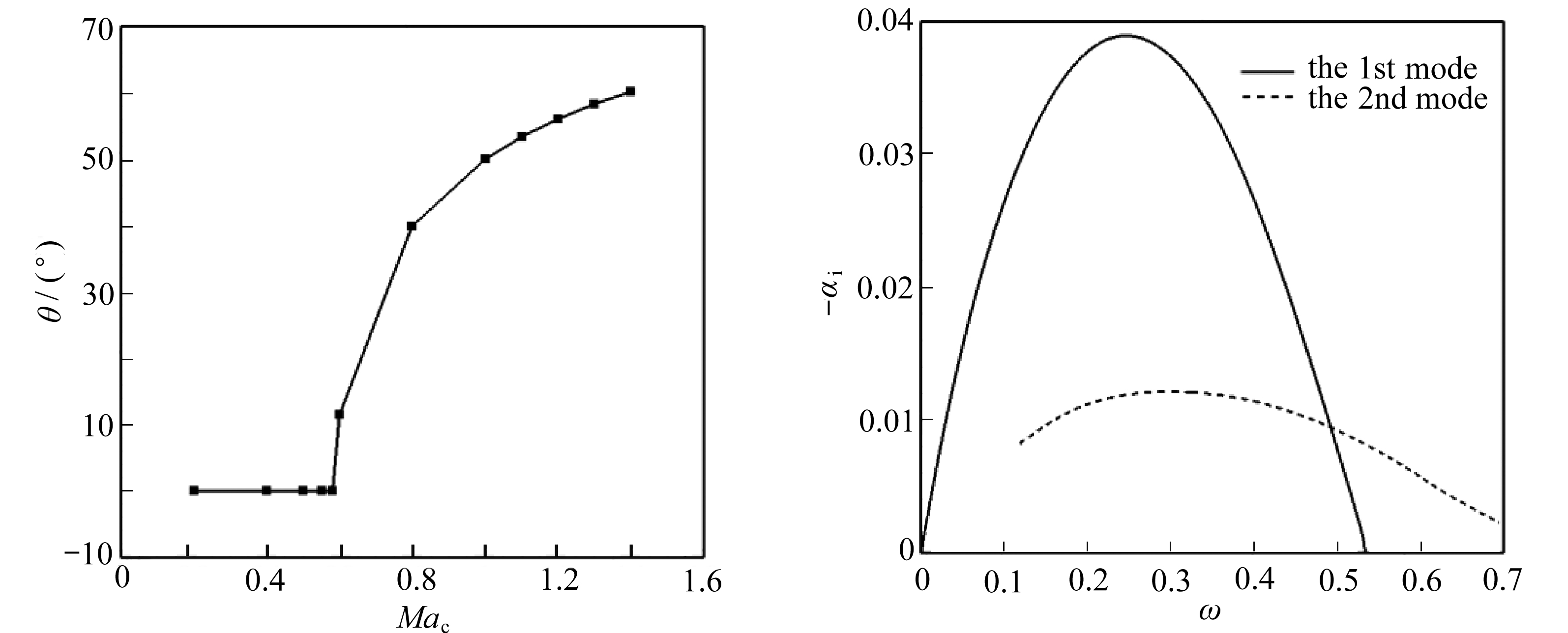Fig. 13 The wave angle of the most unstable Fig. 14 The growth rates of the most unstable wave of the 1st mode changing with wave of the 1st and 2nd modes at the convective Mach number Ma c =1.1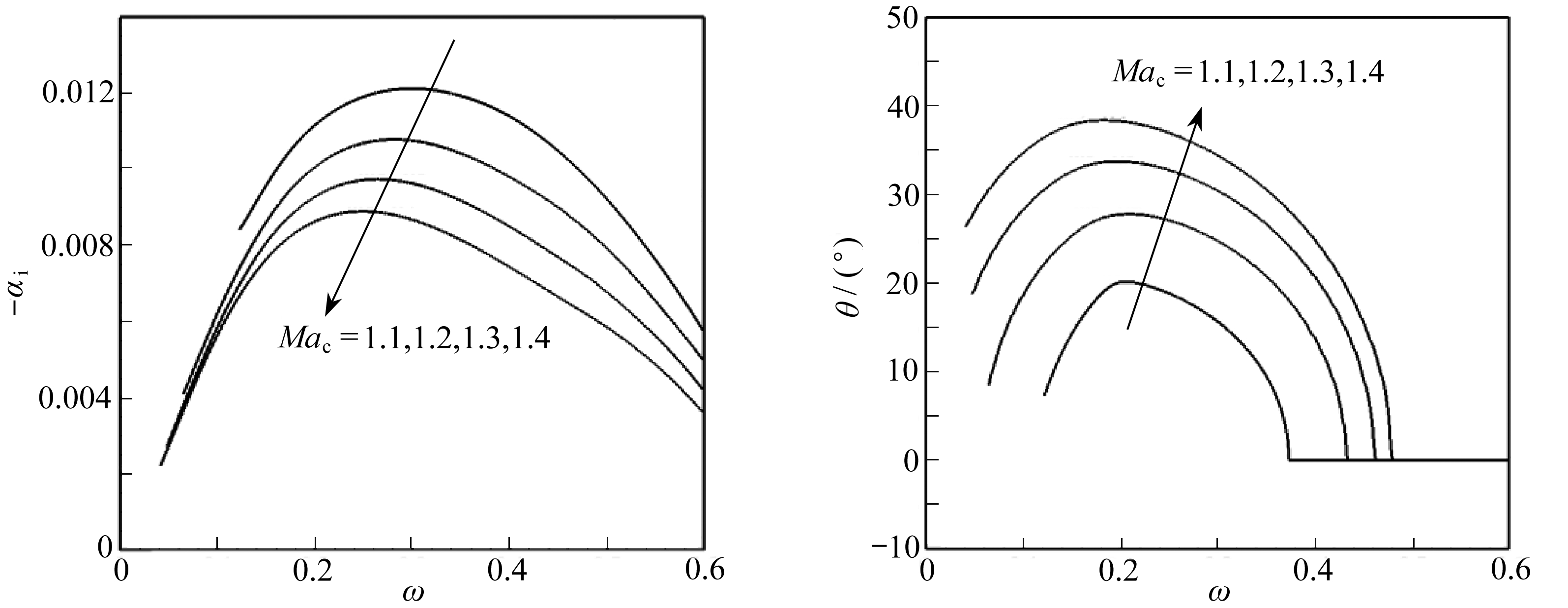Fig. 15 The maximum growth rates of the 2nd Fig. 16 The wave angles of the most unstable wave mode vs. the frequency at different of the 2nd mode vs. the frequency convective Mach numbers at different Mach numbers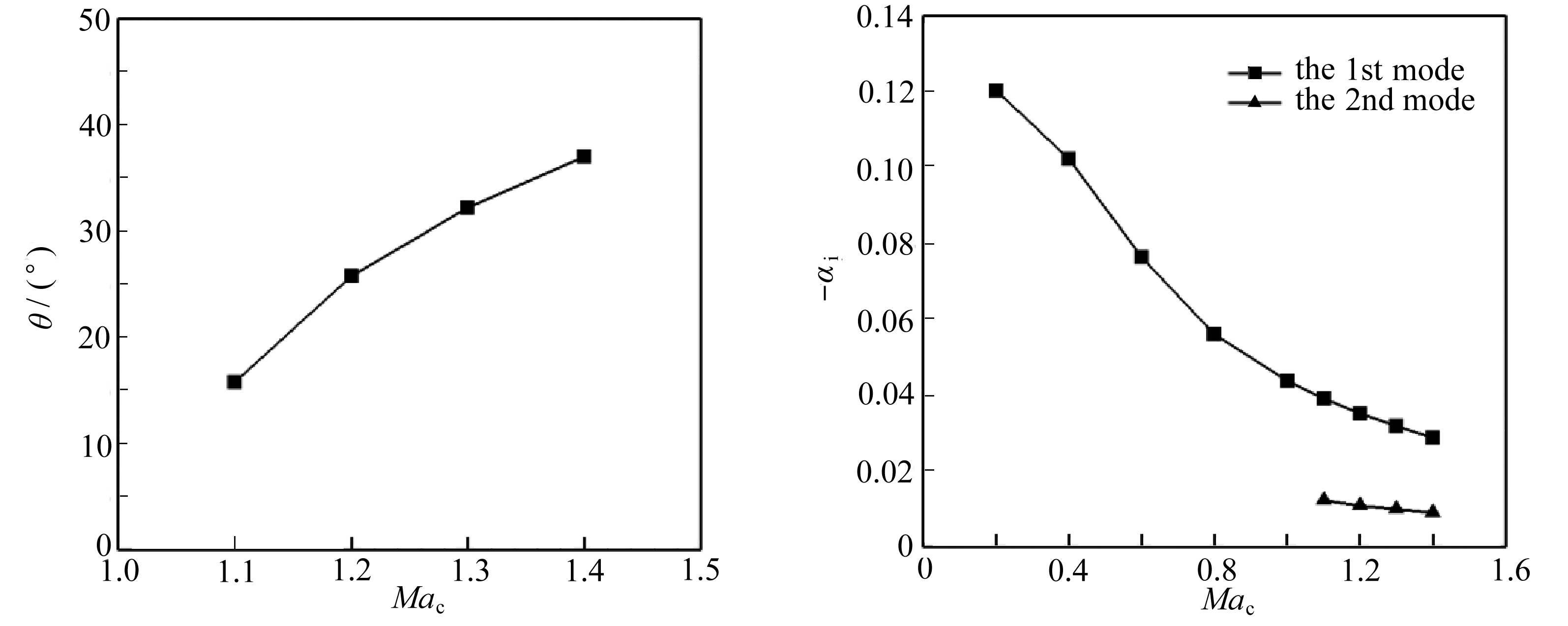Fig. 17 The wave angle of the most unstable wave Fig. 18 The maximum growth rates of the 1st and of the 2nd mode changing with the 2nd modes at different convective convective Mach number Mach numbers

## 3 结 论

 何国强, 秦飞, 魏祥庚, 等. 火箭冲压组合发动机燃烧的若干基础问题研究[J]. 实验流体力学, 2016, 30 (1): 1-14.(HE Guoqiang, QIN Fei, WEi Xianggeng, et al. Investigation of several fundamental combustion problems in rocket-based combined-cycle engines[J]. Journal of Experiments in Fluid Mechanics , 2016, 30 (1): 1-14.(in Chinese))

 李睿劬, 马汉东. 高超声速边界层气动光学研究综述[J]. 气体物理, 2015, 10 (2): 17-28.(LI Ruiqu, MA Handong. Summary on hypersonic boundary-layer aero-optical investigations[J]. Physics of Gases , 2015, 10 (2): 17-28.(in Chinese))

 甘才俊, 熊红亮, 马汉东. 可压缩混合层气动光学效应研究[J]. 计算物理, 2010, 27 (1): 65-72.(GAN Caijun, XIONG Hongliang, MA Handong. Aero-optical effect in compressible mixing layer[J]. Chinese Journal of Computational Physics , 2010, 27 (1): 65-72.(in Chinese))

 杨志洋, 赵磊, 罗纪生. 超音速后掠椭圆柱横流定常涡的不稳定特性及转捩预测[J]. 应用数学和力学, 2017, 38 (8): 853-862.(YANG Zhiyang, ZHAO Lei, LUO Jisheng. Instability and transition prediction of stationary crossflow vortices over supersonic swept elliptic cylinders[J]. Applied Mathematics and Mechanics , 2017, 38 (8): 853-862.(in Chinese))

 MICHALKE A. On spatially growing disturbances in aninviscid shear layer[J]. Journal of Fluid Mechanics , 1965, 23 (3): 521-544.

 BLUMEN W, DRAZIN P G, BILLINGS D F. Shear layer instability of an inviscid compressible fluid: part 2[J]. Journal of Fluid Mechanics , 1975, 71 (2): 305-316.

 JACKSON T L, GROSCH C E. Inviscid spatial stability of a compressible mixing layer[J]. Journal of Fluid Mechanics , 1989, 217 : 609-637.

 罗纪生, 吕祥翠. 超音速混合层稳定性分析及增强混合的研究[J]. 力学学报, 2004, 36 (2): 202-207.(LUO Jisheng, LÜ Xiangcui. Investigation on stability of 3-D supersonic mixing layer and method of enhancing the mixing[J]. ACTA Mechanica Sinica , 2004, 36 (2): 202-207.(in Chinese))

 王强, 傅德薰, 马延文. 可压缩平面混合层稳定性数值计算[J]. 计算物理, 1997, 14 (4/5): 413-416.(WANG Qiang, FU Dexun, MA Yanwen. A numerical investigation of the stability of compressible plane mixing layers[J]. Chinese Journal of Computational Physics , 1997, 14 (4/5): 413-416.(in Chinese))

 杨武兵, 庄逢甘, 沈清. 可压缩混合层流动近十年研究进展[J]. 力学进展, 2008, 38 (1): 62-76.(YANG Wubing, ZHUANG Fenggan, SHEN Qing. Advances in the study of compressible mixing layer in recent ten years[J]. Advances in Mechanics , 2008, 38 (1): 62-76.(in Chinese))

 沈清, 袁湘江, 王强, 等. 可压缩边界层与混合层失稳结构的研究进展及其工程应用[J]. 力学进展, 2012, 42 (3): 252-261.(SHEN Qing, YUAN Xiangjiang, WANG Qiang, et al. Review on the instability structure in compressible boundary layers and mixing layers and its application[J]. Advances in Mechanics , 2012, 42 (3): 252-261.(in Chinese))

 KOZUSKO F, LASSEIGNE D G, GROSH C E, et al. The stability of compressible mixing layers in binary gases[J]. Physics of Fluids , 1996, 8 (7): 1954-1963.

 LEONARDO S, MARCIO T M. Spatial and temporal linear stability analysis of binary compressible shear layer[C]// AIAA 38 th Fluid Dynamics Conference and Exhibit . Seattle, Washington, USA, 2008.

 OKONG N A, BELLAN J. Real gas effects on mean flow and temporal stability of binary-species mixing layers[J]. AIAA Journal , 2003, 41 (12): 2429-2443.

 FEDIOUN I, LARDJANE N. Temporal linear stability analysis of three-dimensional compressible binary shear layers[J]. AIAA Journal , 2005, 43 (1): 111-123.

 MENDONCA M T. Linear stability analysis of binary compressible mixing layers modified by a jet or a wake deficit[C]//52 nd Aerospace Sciences Meeting . 2014: 1444. DOI: 10.2514/6.2014-1444.

 SHIN D S, FERZIGER J H. Linear stability of the reacting mixing layer[J]. AIAA Journal , 1991, 29 (10): 1634-1642.

 DAY M J, REYNOLDS W C, MANSOUR N N. The structure of the compressible reacting mixing layer: Insights from linear stability analysis[J]. Physics of Fluid , 1998, 10 (4): 993-1007.

# Stability Analysis of Binary Gas Mixing Layers

GAO Jun 1 , LI Jia 2 , LIU Fengjun 3 ,SHI Xiaotian 1 , YUAN Xiangjiang 1

(1. China Academy of Aerospace Aerodynamics , Beijing 100074, P . R . China ,2. Foundation Department , Tangshan College , Tangshan , Hebei 063000, P . R . China ; 3. Beijing Power Machinery Institute , Beijing 100074, P . R . China )

Abstract : For the mixing layer flow composed of oxygen and nitrogen, the linear stability analysis was utilized to investigate the stability characteristics. The basic flow profile of the binary gas mixing layers was obtained from the similar solution. The effects of the convective Mach number on the similar solution were firstly investigated. Then, the influences of the convective Mach number, 2D and 3D waves on the stability were studied. The results of linear stability indicate that, the maximum growth rate of the 1st mode is always larger than that of the 2nd mode, and the maximum growth rates of the 1st and 2nd modes are suppressed with the increasing convective Mach number.

Key words: linear stability; mixing layer; binary gas; convective Mach number; similar solution

Foundation item: The National Natural Science Foundation of China(51476152； 11302213)

ⓒ 应用数学和力学编委会，ISSN 1000-0887

* 收稿日期 2018-02-27；

DOI: 10.21656/1000-0887.390064

①引用本文 / Cite this paper: 高军, 李佳, 刘凤君, 时晓天, 袁湘江. 二元气体混合层的稳定性分析[J]. 应用数学和力学, 2018, 39 (9): 1030-1042.GAO Jun, LI Jia, LIU Fengjun, SHI Xiaotian, YUAN Xiangjiang. Stability analysis of binary gas mixing layers[J]. Applied Mathematics and Mechanics , 2018, 39 (9): 1030-1042.